Last update:
April 29, 2017, 01:05

## Triangles, Circles and Vectors: A Brief Reference for Programmers

##### By rolliebollocks

If you ever sat in algebra class wondering what any of it was good for, you are in for a rude shock. Algebra is a necessary skill for any programmer, and programmers of all fields need to be able to work with it to be effective.

#### Pythagoras

##### a^2 + b^2 = c^2 and Soh Cah Toa

According to the Pythagorean theorem, the hypotenuse of any right triangle is equal to the square root of the sum of its sides squared. This means that if we root the sums of the squares of a&b, we will have the length of c, the hypotenuse.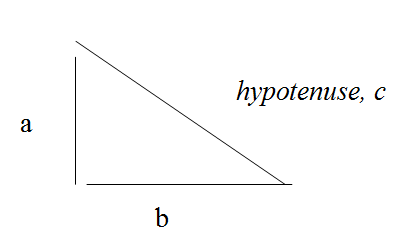This is also relevant to vector mathematics, which is an integral part of game programming. A vector is a mathematical term for a geometrical object called a ray: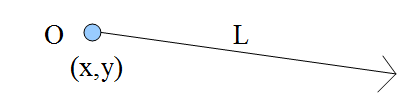Our ray has an origin at x and y, and a length or magnitude of L. If we were to treat L like a hypotenuse, we could draw the remaining portion of the triangle like so: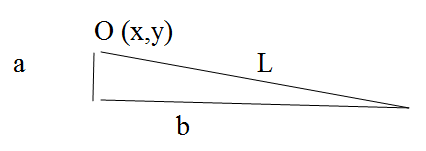So, let's say we know that L has a magnitude of 5. And we also know that L is at some angle to B, and we'll assume it's 30 degrees.

In order to find the location of our vector at its current magnitude, let's first redraw the vector: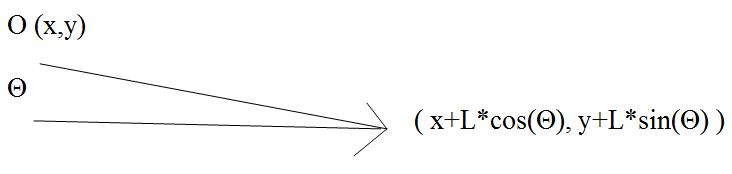This method is identical to the Soh Cah Toa conversions which deal with right triangles:

sin T = Opposite/Hypotenuse

It is important to remember that the x component is related to the cosine, and that the y component is related to the sin. Mix these at your own peril.

By utilizing this information, we can determine the angle per step in time. It is important to remember that a vector is an object moving through space in a single direction. Every step it takes in both x and y directions is considered its unit vector. So for each time step, we move the unit vector's x component and y component.

To determine the unit vector, we divide the "opposite" over the "hypotenuse" to get the sine, or y angle, and the "adjacent" over the "hypotenuse" to get the x angle/cosine.

So if we're dealing with a 3:4:5 right triangle, then:

UnitVector X = 4/5

UnitVector Y = 3/5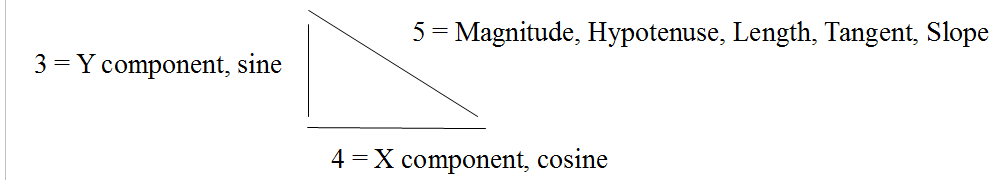This pertains to Circles

The unit circle is a circle with a radius of 1: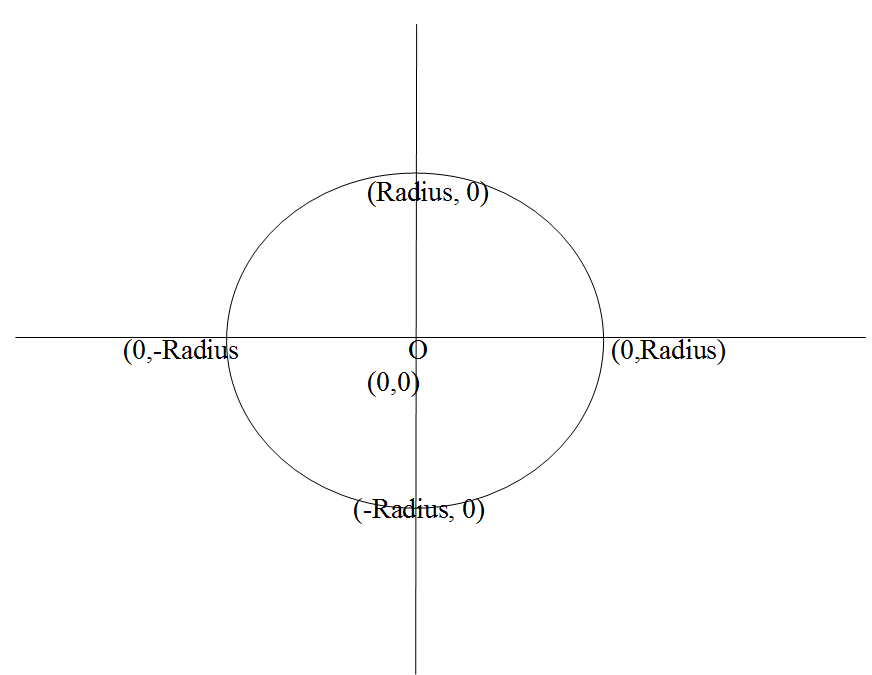The formula for any circle is given as:

This means that the square of the radius is in direct proportion to the sums of the squares of the x and y values. It is worth noting that this equation has the exact same structure as the Pythagorean Theorem a^2 + b^2 = c^2.

#### In Scales Now

If we want a circle twice the size of the unit circle, we can either multiply the unit circle's radius by two, or we can divide the x and y components by two. To scale it by three we would do the same thing. For ease, it's usually better to adjust the x and y components to the size of the radius than vice versa. We will get to that tactic in just a moment.

Remember when we were talking about vectors? I mentioned that the vector travels at a specific trajectory that can be determined by solving for the unit vector. When you scale a vector in this manner, you scale it through multiples of the unit vector, which is a temporal dimension expressed in time, as in: distance = rate * time. The "time" is a scalar multiple of the vector at some step from the vector's origin. At time step zero, the result of the vector is its origin. At one, it is the origin plus the unit vector. At two, it is the origin plus two times the unit vector. We can think of a vector like time line; each step is a scalar multiple of the unit vector in Cartesian coordinates beginning at the origin; a thing moving through space in a constant direction.

#### Colliding Circles

So we have particle 1 at 17,35 on our grid, and we have particle 2 at 90,45. If particle 2 has a radius of 17, how big does particle 1 have to be to register a collision?

To determine how far the x and y components are from each other, we first subtract them from one another:

17-90 = -73

35-45= -10

In geometric terms, we now have this: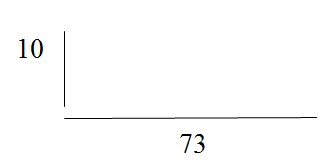In order to get the actual distance we will solve using Pythagoras again, which will cause our negative values to lose their sign (and also their direction in relation to each other).

So...

```10^2 = 100
73^2 = 5329
+__________
5429
Distance = Sqrt(5429) = 73.68```

Now we simply subtract the radius of particle 2 from the distance:

73.68 – 17 = 56.68

And now we know how big our other particle's radius must be in order to collide with the first particle. In short, we get the distance by taking the square root of the sums of the squares of the differences of the x's and the y's. Using this formula is a good way to approximate any collision, though there are more precise methods. If you wanted to use it on two sprites, you could create a function like this:

```#include once "fbgfx.bi"
declare function iCollide ( img1 as fb.image ptr, img2 as fb.image ptr, _
x1 as single, y1 as single, x2 as single, y2 as single ) as integer

function iCollide ( img1 as fb.image ptr, img2 as fb.image ptr, _
x1 as single, y1 as single, _
x2 as single, y2 as single ) as integer

'X is width, Y is height

'Get data from image pointer
dim as integer w1 = img1->width
dim as integer w2 = img2->width
dim as integer h1 = img1->height
dim as integer h2 = img2->height

'Find the center
dim as single center1x = w1/2
dim as single center1y = h1/2
dim as single center2x = w2/2
dim as single center2y = h2/2

'Add the center to the x and y values of the
'image's location. This is the position of the
'center of our sprite. The radius is equal to pythagoras
'hypotenuse of x and y components of the center.

dim as single pos1x = x1 + center1x
dim as single pos1y = y1 + center1y
dim as single pos2x = x2 + center2x
dim as single pos2y = y2 + center2y

dim as single radius1 = sqr((w1*h1)/3.1459)
dim as single radius2 = sqr((w2*h2)/3.1459)

'Employ distance forumla

dim as single distance = sqr( (pos1x-pos2x)^2 + (pos1y-pos2y)^2 )

return -1 'True
else
return 0 'False
endif
end function
'Begin Example
RANDOMIZE
screen 19,32
Const as integer screen_x = 800
Const as integer screen_y = 600
dim as fb.image ptr img1 = imagecreate(100,100,&hff00ff)
dim as fb.image ptr img2 = imagecreate(145,145,&hff00ff)
circle img1, (50,50),45,&hffffff,,,,f
circle img2, (72,72),70,&hffffff,,,,f
dim as single x1,y1,x2,y2
x1=rnd*screen_x
y1=rnd*screen_y
x2=rnd*screen_x
y2=rnd*screen_y
do

screenlock
cls
Put (x1,y1),img1,pset
Put (x2,y2),img2,pset
Locate 1,1: ? "Collision: ", iCollide(img1,img2, x1,y1,x2,y2)
screenunlock

sleep

x1=rnd*screen_x-100
y1=rnd*screen_y-100
x2=rnd*screen_x-100
y2=rnd*screen_y-100
loop until multikey(fb.sc_escape)```
Powered by CMSimple | CMSimple Legal Notices | (X)html | css | Login | Template-Design: Lars Ellmauer | Template-Modified: Imortisoft ISD